# Types and Principles of Hall Elements

#03 Basic Knowledge of Magnetic Sensor

A Hall element is an element that utilizes the Hall effect to produce an output voltage (VH) proportional to a magnetic field (B) applied perpendicular to its surface. The output voltage is the differential output across terminals 2 and 4 in Figure 1a. The relationship between the output voltage and the magnetic field applied to the Hall element can be seen in Figure 1b.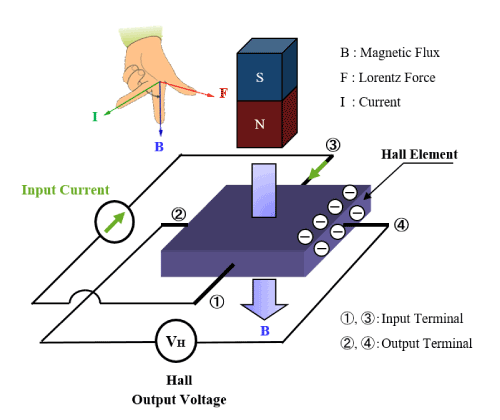Figure 1a. Principle operation of a Hall element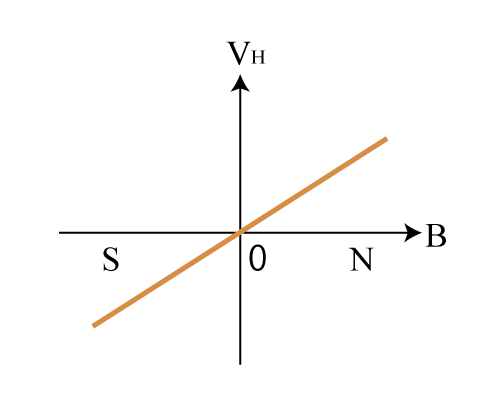Figure 1b. Output voltage characteristics of a Hall element

## Drive System

Hall elements are available in two drive modes: constant current drive and constant voltage drive. The temperature characteristic of the Hall element is dependent upon the drive mode.

### Constant current drive

When a constant current (IC) is input into terminals 1 and 3, the output voltage at terminals 2 and 4 can be expressed by the following equation:
VH ＝ RH・(1 / d)・IC・B
Where RH is the Hall coefficient, B is the magnetic flux density, and d is the thickness of the semiconductor film. The Hall coefficient RH is defined as follows using electron charge (e) and carrier concentration (n):
RH = 1 / (e・n）
The temperature characteristic of the output voltage with constant current drive is determined by the temperature characteristic of the Hall coefficient.

### Constant voltage drive

When a fixed voltage (VC) is applied across terminals 1 and 3, the output voltage (VH) at terminals 2 and 4 can be expressed by the following equation:
VH = μH・ (W / L) ・VC・B
Where μH is the electron mobility, W is the width of the element surface, and L is the length of the element surface. The temperature characteristic of the output voltage of an element with constant voltage drive is determined by the temperature characteristic of μH.Figure 2. VH temperature characteristics of constant current drive and constant voltage drive (Example: Ultra high sensitivity Hall element, InSb, B=50mT)

## Offset Voltage

Hall elements may generate an output voltage even when no magnetic field is applied, called an offset voltage (or unbalanced voltage). This offset voltage is generated due to unintended variations in the manufacturing process and the offset voltage is also temperature dependent.

The offset component of the Hall element can be represented by a bridge circuit of four resistance values as shown in Figure 3a.

If all four resistors are equal, the offset voltage will be zero. When an offset voltage is present, the output voltage characteristic will be shifted as shown in Figure 3b.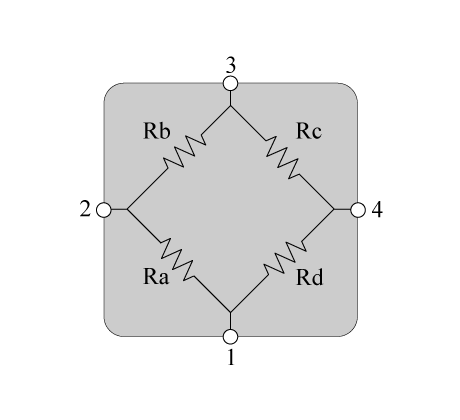Figure 3a. Equivalent circuit of a Hall element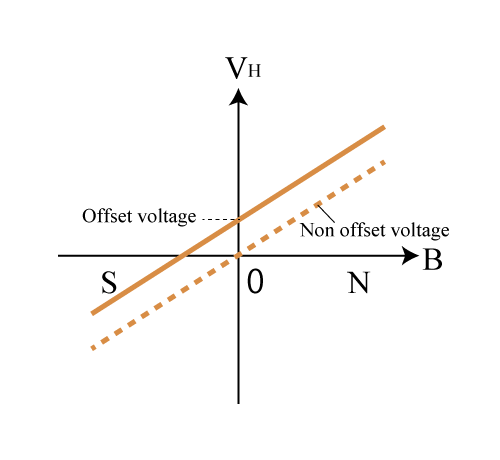Figure 3b. Output voltage with and without an offset voltage

## Hall Element Types

AKM provides three types of Hall elements composed of different semiconductor film materials: supersensitive Hall elements made of indium antimonide (InSb), high sensitivity Hall elements made of indium arsenide (InAs), and low drift Hall elements made of gallium arsenide (GaAs).
The primary semiconductor materials currently used as Hall elements are shown in Table 1 along with their unique characteristics.

Although silicon (Si) is one of the common Hall element materials, it is not used as a Hall element in the product lines shown in the table because of its low sensitivity. Silicon is used in Hall ICs. The sensitivity of the Hall element is proportional to the mobility of semiconductor material. Temperature characteristics are affected by various factors, but they are primarily determined by the band gap.
Temperature characteristics and sensitivity of the output voltage differ based on the material of the Hall element. The user should choose a Hall element that is suitable for their specific application. The features of the various Hall element types are as follows: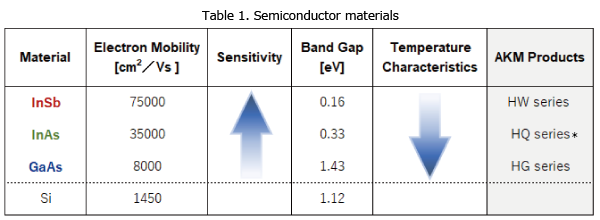*Also containing Ga,Sb etc. as materials.

### Features

・HW series: This is the most sensitive among the three Hall element types.
・HG series: This has the most stable temperature characteristic among the three Hall element types.
・HQ series: This Hall element has a good balance of sensitivity and temperature characteristics.

Figure 4 shows the output voltage characteristics of the 3 types of Hall elements using constant current drive with the same control current (IC=5mA).

Figure 5 shows the output voltage characteristics of the 3 types of Hall elements using constant voltage drive with the same control voltage (VC=1V).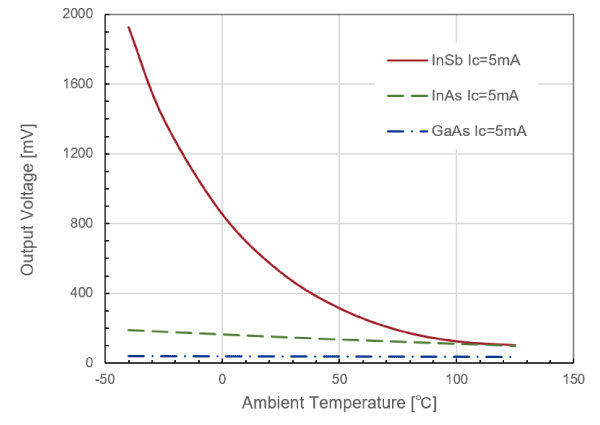Figure 4. Output voltage with constant current drive (IC=5mA, B=50mT)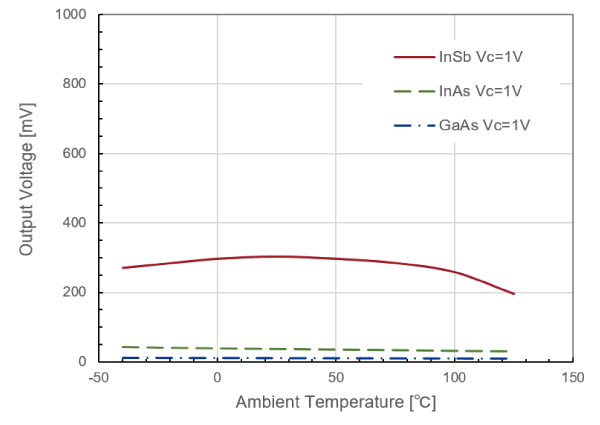Figure 5. Output voltage with constant voltage drive (VC=1V, B=50mT)